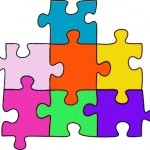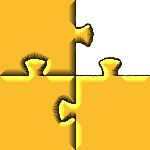## How do I build a linear equation jigsaw puzzle?Unlike traditional “picture” jigsaw puzzles, linear equation jigsaw puzzles are largely blank. There are seldom patterns or background images to guide you as you put the pieces together. Although traditionally rectangular, some puzzles are designed to take on unexpected shapes when completed. However, these “shaped” puzzles are not usually sold with obvious clues that will allow the puzzle builder to construct the puzzle using only the goal of a particular shape. There are no short-cuts, cheat-sheets, or ways to avoid solving the equations. If you want to build the puzzle, you must first solve the equations printed on the puzzle pieces – they alone hold the keys to putting the puzzle together. If you are new to linear equation jigsaw puzzles, and need some help getting starting, read “How to Solve a Linear Equation Jigsaw Puzzle“.

## Are linear equation jigsaw puzzles only for classroom use?Puzzles are enjoyed by everyone from grandma to your toddler. Classrooms certainly don’t have exclusive rights over them. While perfect for building class spirit and developing team work skills within the classroom environment, linear equation jigsaw puzzles are even more useful at home.

If you home school your children, integrate the puzzles in your home school lesson plan to spice up traditional algebra lessons. Parents with children who show reluctance to do their algebra homework can encourage an interest in the subject by introducing these puzzles as part of a reward system. For example, for every 3 traditional equation worksheets completed, the child could earn the opportunity to complete a linear equation jigsaw puzzle instead of a worksheet.

Does your family enjoy building puzzles together? Take it to the next level by completing a linear equation jigsaw puzzle as a family. This type of family activity helps encourage an appreciation for mathematics, and teaches children that the topics they deal with “in school” are not for exclusive use in school.

## Solving Linear Equations is the Game to PlayBad experiences with linear equations can brand algebra as the subject to hate. Many a middle-schooler has adopted this negative attitude during the early stages of exposure to algebra. As countless high school mathematics teachers will attest, changing this perception of algebra as being a “boring and difficult” subject is not easy. But solving linear equations need not be the doorway to mathematical doom and darkness. Repetition is certainly necessary to develop problem-solving skill, but the unexciting repetition that usually kills any interest in algebra can be presented as something fun and challenging. How this goal is achieved is limited only by the imagination of the teacher. A favorite for me is to present the activity of solving linear equations as a jigsaw puzzle. The puzzle may be offered as an individual or a team challenge, depending on the objectives of the teacher.

Linear equation jigsaw puzzles are the game-players’ alternative to solving pages of equations. These puzzles take advantage of all the skill-developing attributes of puzzle building, but do this on top of developing algebraic problem-solving skills. Before the second piece of the puzzle can be laid, a linear equation must be solved. While some students may be hesitant to embrace the challenge of a page of linear equations begging solutions, few will back down from the chance to build a puzzle.

The rate at which the puzzle can be built is primarily determined by the speed at which the student can solve the linear equations. The linear equation jigsaw puzzle therefore has the potential to serve as an informal speed test, but teachers should be cautioned against using these puzzles for formal tests. Since jigsaw puzzle building depends on the hand-eye co-ordination and the spatial perception of the students, timed test results may be indicative of more than just the student’s ability to solve linear equations.